# 26.1: Organic Compounds and Structures: An Overview

$$\newcommand{\vecs}{\overset { \rightharpoonup} {\mathbf{#1}} }$$ $$\newcommand{\vecd}{\overset{-\!-\!\rightharpoonup}{\vphantom{a}\smash {#1}}}$$$$\newcommand{\id}{\mathrm{id}}$$ $$\newcommand{\Span}{\mathrm{span}}$$ $$\newcommand{\kernel}{\mathrm{null}\,}$$ $$\newcommand{\range}{\mathrm{range}\,}$$ $$\newcommand{\RealPart}{\mathrm{Re}}$$ $$\newcommand{\ImaginaryPart}{\mathrm{Im}}$$ $$\newcommand{\Argument}{\mathrm{Arg}}$$ $$\newcommand{\norm}{\| #1 \|}$$ $$\newcommand{\inner}{\langle #1, #2 \rangle}$$ $$\newcommand{\Span}{\mathrm{span}}$$ $$\newcommand{\id}{\mathrm{id}}$$ $$\newcommand{\Span}{\mathrm{span}}$$ $$\newcommand{\kernel}{\mathrm{null}\,}$$ $$\newcommand{\range}{\mathrm{range}\,}$$ $$\newcommand{\RealPart}{\mathrm{Re}}$$ $$\newcommand{\ImaginaryPart}{\mathrm{Im}}$$ $$\newcommand{\Argument}{\mathrm{Arg}}$$ $$\newcommand{\norm}{\| #1 \|}$$ $$\newcommand{\inner}{\langle #1, #2 \rangle}$$ $$\newcommand{\Span}{\mathrm{span}}$$$$\newcommand{\AA}{\unicode[.8,0]{x212B}}$$

##### Learning Objectives
• To recognize the composition and properties typical of organic and inorganic compounds.
• To identify and name simple (straight-chain) alkanes given formulas and write formulas for straight-chain alkanes given their names.
• Write condensed structural formulas for alkanes given complete structural formulas.
• Draw line-angle formulas given structural formulas.
• To name alkanes by the IUPAC system and write formulas for alkanes given IUPAC names
• To describe functional groups and explain why they are useful in the study of organic chemistry.

Scientists of the 18th and early 19th centuries studied compounds obtained from plants and animals and labeled them organic because they were isolated from “organized” (living) systems. Compounds isolated from nonliving systems, such as rocks and ores, the atmosphere, and the oceans, were labeled inorganic. For many years, scientists thought organic compounds could be made by only living organisms because they possessed a vital force found only in living systems. The vital force theory began to decline in 1828, when the German chemist Friedrich Wöhler synthesized urea from inorganic starting materials. He reacted silver cyanate (AgOCN) and ammonium chloride (NH4Cl), expecting to get ammonium cyanate (NH4OCN). What he expected is described by the following equation.

$AgOCN + NH_4Cl \rightarrow AgCl + NH_4OCN \label{Eq1}$

Instead, he found the product to be urea (NH2CONH2), a well-known organic material readily isolated from urine. This result led to a series of experiments in which a wide variety of organic compounds were made from inorganic starting materials. The vital force theory gradually went away as chemists learned that they could make many organic compounds in the laboratory.

Today organic chemistry is the study of the chemistry of the carbon compounds, and inorganic chemistry is the study of the chemistry of all other elements. It may seem strange that we divide chemistry into two branches—one that considers compounds of only one element and one that covers the 100-plus remaining elements. However, this division seems more reasonable when we consider that of tens of millions of compounds that have been characterized, the overwhelming majority are carbon compounds.

##### Note

The word organic has different meanings. Organic fertilizer, such as cow manure, is organic in the original sense; it is derived from living organisms. Organic foods generally are foods grown without synthetic pesticides or fertilizers. Organic chemistry is the chemistry of compounds of carbon.

Carbon is unique among the other elements in that its atoms can form stable covalent bonds with each other and with atoms of other elements in a multitude of variations. The resulting molecules can contain from one to millions of carbon atoms. We previously surveyed organic chemistry by dividing its compounds into families based on functional groups. We begin with the simplest members of a family and then move on to molecules that are organic in the original sense—that is, they are made by and found in living organisms. These complex molecules (all containing carbon) determine the forms and functions of living systems and are the subject of biochemistry.

Organic compounds, like inorganic compounds, obey all the natural laws. Often there is no clear distinction in the chemical or physical properties among organic and inorganic molecules. Nevertheless, it is useful to compare typical members of each class, as in Table $$\PageIndex{1}$$.

Table $$\PageIndex{1}$$: General Contrasting Properties and Examples of Organic and Inorganic Compounds
Organic Hexane Inorganic NaCl
low melting points −95°C   high melting points 801°C
low boiling points 69°C high boiling points 1,413°C
low solubility in water; high solubility in nonpolar solvents insoluble in water; soluble in gasoline greater solubility in water; low solubility in nonpolar solvents soluble in water; insoluble in gasoline
flammable highly flammable nonflammable nonflammable
aqueous solutions do not conduct electricity nonconductive aqueous solutions conduct electricity conductive in aqueous solution
exhibit covalent bonding covalent bonds exhibit ionic bonding ionic bonds

Keep in mind, however, that there are exceptions to every category in this table. To further illustrate typical differences among organic and inorganic compounds, Table $$\PageIndex{1}$$ also lists properties of the inorganic compound sodium chloride (common table salt, NaCl) and the organic compound hexane (C6H14), a solvent that is used to extract soybean oil from soybeans (among other uses). Many compounds can be classified as organic or inorganic by the presence or absence of certain typical properties, as illustrated in Table $$\PageIndex{1}$$.

## Hydrocarbons

We begin our study of organic chemistry with the hydrocarbons, the simplest organic compounds, which are composed of carbon and hydrogen atoms only. As we noted, there are several different kinds of hydrocarbons. They are distinguished by the types of bonding between carbon atoms and the properties that result from that bonding. Hydrocarbons with only carbon-to-carbon single bonds (C–C) and existing as a continuous chain of carbon atoms also bonded to hydrogen atoms are called alkanes (or saturated hydrocarbons). Saturated, in this case, means that each carbon atom is bonded to four other atoms (hydrogen or carbon)—the most possible; there are no double or triple bonds in the molecules.

##### Note

The word saturated has the same meaning for hydrocarbons as it does for the dietary fats and oils: the molecule has no carbon-to-carbon double bonds (C=C).

We previously introduced the three simplest alkanes—methane (CH4), ethane (C2H6), and propane (C3H8) and they are shown again in Figure $$\PageIndex{1}$$.Figure $$\PageIndex{1}$$: The Three Simplest Alkanes

The flat representations shown do not accurately portray bond angles or molecular geometry. Methane has a tetrahedral shape that chemists often portray with wedges indicating bonds coming out toward you and dashed lines indicating bonds that go back away from you. An ordinary solid line indicates a bond in the plane of the page. Recall that the VSEPR theory correctly predicts a tetrahedral shape for the methane molecule (Figure $$\PageIndex{2}$$).Figure $$\PageIndex{2}$$: The Tetrahedral Methane Molecule

Methane (CH4), ethane (C2H6), and propane (C3H8) are the beginning of a series of compounds in which any two members in a sequence differ by one carbon atom and two hydrogen atoms—namely, a CH2 unit. The first 10 members of this series are given in Table $$\PageIndex{2}$$.

Table $$\PageIndex{2}$$: The First 10 Straight-Chain Alkanes
Name Molecular Formula (CnH2n + 2) Condensed Structural Formula Number of Possible Isomers
methane CH4 CH4
ethane C2H6 CH3CH3
propane C3H8 CH3CH2CH3
butane C4H10 CH3CH2CH2CH3 2
pentane C5H12 CH3CH2CH2CH2CH3 3
hexane C6H14 CH3CH2CH2CH2CH2CH3 5
heptane C7H16 CH3CH2CH2CH2CH2CH2CH3 9
octane C8H18 CH3CH2CH2CH2CH2CH2CH2CH3 18
nonane C9H20 CH3CH2CH2CH2CH2CH2CH2CH2CH3 35
decane C10H22 CH3CH2CH2CH2CH2CH2CH2CH2CH2CH3 75

Consider the series in Figure $$\PageIndex{3}$$. The sequence starts with C3H8, and a CH2 unit is added in each step moving up the series. Any family of compounds in which adjacent members differ from each other by a definite factor (here a CH2 group) is called a homologous series. The members of such a series, called homologs, have properties that vary in a regular and predictable manner. The principle of homology gives organization to organic chemistry in much the same way that the periodic table gives organization to inorganic chemistry. Instead of a bewildering array of individual carbon compounds, we can study a few members of a homologous series and from them deduce some of the properties of other compounds in the series.Figure $$\PageIndex{3}$$: Members of a Homologous Series. Each succeeding formula incorporates one carbon atom and two hydrogen atoms more than the previous formula.

The principle of homology allows us to write a general formula for alkanes: CnH2n + 2. Using this formula, we can write a molecular formula for any alkane with a given number of carbon atoms. For example, an alkane with eight carbon atoms has the molecular formula C8H(2 × 8) + 2 = C8H18.

We use several kinds of formulas to describe organic compounds. A molecular formula shows only the kinds and numbers of atoms in a molecule. For example, the molecular formula C4H10 tells us there are 4 carbon atoms and 10 hydrogen atoms in a molecule, but it doesn’t distinguish between butane and isobutane. A structural formula shows all the carbon and hydrogen atoms and the bonds attaching them. Thus, structural formulas identify the specific isomers by showing the order of attachment of the various atoms. Unfortunately, structural formulas are difficult to type/write and take up a lot of space. Chemists often use condensed structural formulas to alleviate these problems. The condensed formulas show hydrogen atoms right next to the carbon atoms to which they are attached, as illustrated for butane: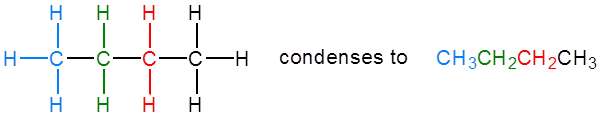The ultimate condensed formula is a line-angle formula, in which carbon atoms are implied at the corners and ends of lines, and each carbon atom is understood to be attached to enough hydrogen atoms to give each carbon atom four bonds. For example, we can represent pentane (CH3CH2CH2CH2CH3) and isopentane [(CH3)2CHCH2CH3] as follows:##### Note

Parentheses in condensed structural formulas indicate that the enclosed grouping of atoms is attached to the adjacent carbon atom.

## Nomenclature

As noted in Table $$\PageIndex{2}$$:, the number of isomers increases rapidly as the number of carbon atoms increases. There are 3 pentanes, 5 hexanes, 9 heptanes, and 18 octanes. It would be difficult to assign unique individual names that we could remember. A systematic way of naming hydrocarbons and other organic compounds has been devised by the International Union of Pure and Applied Chemistry (IUPAC). These rules, used worldwide, are known as the IUPAC System of Nomenclature. (Some of the names we used earlier, such as isobutane, isopentane, and neopentane, do not follow these rules and are called common names.) A stem name (Table $$\PageIndex{3}$$) indicates the number of carbon atoms in the longest continuous chain (LCC). Atoms or groups attached to this carbon chain, called substituents, are then named, with their positions indicated by numbers. For now, we will consider only those substituents called alkyl groups.

Table $$\PageIndex{3}$$: Stems That Indicate the Number of Carbon Atoms in Organic Molecules
Stem Number
meth- 1
eth- 2
prop- 3
but- 4
pent- 5
hex- 6
hept- 7
oct- 8
non- 9
dec- 10

An alkyl group is a group of atoms that results when one hydrogen atom is removed from an alkane. The group is named by replacing the -ane suffix of the parent hydrocarbon with -yl. For example, the CH3 group derived from methane (CH4) results from subtracting one hydrogen atom and is called a methyl group. The alkyl groups we will use most frequently are listed in Table $$\PageIndex{4}$$. Alkyl groups are not independent molecules; they are parts of molecules that we consider as a unit to name compounds systematically.

Table $$\PageIndex{4}$$: Common Alkyl Groups
Parent Alkane Alkyl Group Condensed Structural Formula
methanemethylCH3
ethane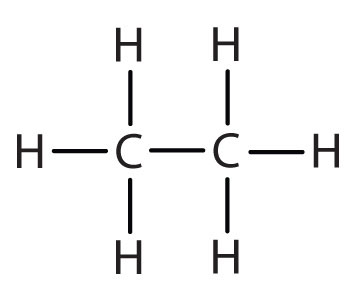ethyl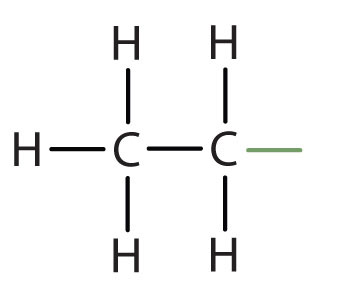CH3CH2
propane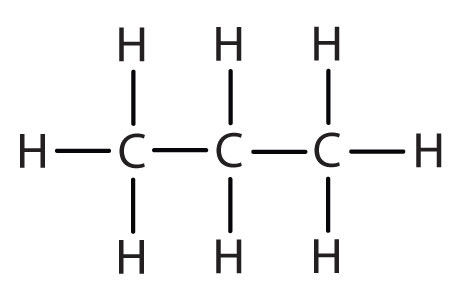propylCH3CH2CH2
isopropyl(CH3)2CH–
butanebutyl*CH3CH2CH2CH2
*There are four butyl groups, two derived from butane and two from isobutane. We will introduce the other three where appropriate.

Simplified IUPAC rules for naming alkanes are as follows (demonstrated in Example $$\PageIndex{1}$$).

1. Name alkanes according to the LCC of carbon atoms in the molecule (rather than the total number of carbon atoms). This LCC, considered the parent chain, determines the base name, to which we add the suffix -ane to indicate that the molecule is an alkane.
2. If the hydrocarbon is branched, number the carbon atoms of the LCC. Numbers are assigned in the direction that gives the lowest numbers to the carbon atoms with attached substituents. Hyphens are used to separate numbers from the names of substituents; commas separate numbers from each other. (The LCC need not be written in a straight line; for example, the LCC in the following has five carbon atoms.)1. Place the names of the substituent groups in alphabetical order before the name of the parent compound. If the same alkyl group appears more than once, the numbers of all the carbon atoms to which it is attached are expressed. If the same group appears more than once on the same carbon atom, the number of that carbon atom is repeated as many times as the group appears. Moreover, the number of identical groups is indicated by the Greek prefixes di-, tri-, tetra-, and so on. These prefixes are not considered in determining the alphabetical order of the substituents. For example, ethyl is listed before dimethyl; the di- is simply ignored. The last alkyl group named is prefixed to the name of the parent alkane to form one word.

When these rules are followed, every unique compound receives its own exclusive name. The rules enable us to not only name a compound from a given structure but also draw a structure from a given name. The best way to learn how to use the IUPAC system is to put it to work, not just memorize the rules. It’s easier than it looks.

##### Example $$\PageIndex{1}$$

Name each compound.

1.2.3.Solution

1. The LCC has five carbon atoms, and so the parent compound is pentane (rule 1). There is a methyl group (rule 2) attached to the second carbon atom of the pentane chain. The name is therefore 2-methylpentane.
2. The LCC has six carbon atoms, so the parent compound is hexane (rule 1). Methyl groups (rule 2) are attached to the second and fifth carbon atoms. The name is 2,5-dimethylhexane.
3. The LCC has eight carbon atoms, so the parent compound is octane (rule 1). There are methyl and ethyl groups (rule 2), both attached to the fourth carbon atom (counting from the right gives this carbon atom a lower number; rule 3). The correct name is thus 4-ethyl-4-methyloctane.
##### Exercise $$\PageIndex{1}$$

Name each compound.

1.2.3.##### Example $$\PageIndex{2}$$

Draw the structure for each compound.

1. 2,3-dimethylbutane
2. 4-ethyl-2-methylheptane

Solution

1. The parent chain is butane, indicating four carbon atoms in the LCC.Then add the groups at their proper positions. You can number the parent chain from either direction as long as you are consistent; just don’t change directions before the structure is done. The name indicates two methyl (CH3) groups, one on the second carbon atom and one on the third.Finally, fill in all the hydrogen atoms, keeping in mind that each carbon atom must have four bonds.2. Adding the groups at their proper positions givesFilling in all the hydrogen atoms gives the following condensed structural formulas:Note that the bonds (dashes) can be shown or not; sometimes they are needed for spacing.

##### Exercise $$\PageIndex{2}$$

Draw the structure for each compound.

1. 4-ethyloctane
2. 3-ethyl-2-methylpentane
3. 3,3,5-trimethylheptane

## Functional Groups

Functional groups are atoms or small groups of atoms (two to four) that exhibit a characteristic reactivity. A particular functional group will almost always display its characteristic chemical behavior when it is present in a compound. Because of their importance in understanding organic chemistry, functional groups have characteristic names that often carry over in the naming of individual compounds incorporating specific groups. In our study of organic chemistry, it will become extremely important to be able to quickly recognize the most common functional groups, because they are the key structural elements that define how organic molecules react. For now, we will only worry about drawing and recognizing each functional group, as depicted by Lewis and line structures. Much of the remainder of your study of organic chemistry will be taken up with learning about how the different functional groups tend to behave in organic reactions.

Previously, we considered several kinds of hydrocarbons. Now we examine some of the many organic compounds that contain functional groups. We first introduced the idea of the functional group, a specific structural arrangement of atoms or bonds that imparts a characteristic chemical reactivity to the molecule. If you understand the behavior of a particular functional group, you will know a great deal about the general properties of that class of compounds. In this chapter, we make a brief yet systematic study of some of organic compound families. Each family is based on a common, simple functional group that contains an oxygen atom or a nitrogen atom. Some common functional groups are listed in Table $$\PageIndex{5}$$ and a more comprehensive list if found here.

Table $$\PageIndex{5}$$: Selected Organic Functional Groups
Name of Family General Formula Functional Group Suffix*
alkane RH none -ane
alkene R2C=CR2-ene
alkyne RC≡CR –C≡C– -yne
alcohol ROH –OH -ol
thiol RSH –SH -thiol
ether ROR –O– ether
aldehyde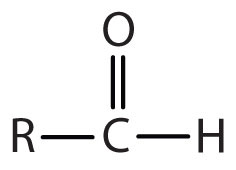-al
ketone-one
carboxylic acid-oic acid
*Ethers do not have a suffix in their common name; all ethers end with the word ether.

Summary

• Simple alkanes exist as a homologous series, in which adjacent members differ by a CH2 unit.
• Condensed chemical formulas show the hydrogen atoms (or other atoms or groups) right next to the carbon atoms to which they are attached.
• Line-angle formulas imply a carbon atom at the corners and ends of lines. Each carbon atom is understood to be attached to enough hydrogen atoms to give each carbon atom four bonds.
• Alkanes have both common names and systematic names, specified by IUPAC.
• The functional group, a structural arrangement of atoms and/or bonds, is largely responsible for the properties of organic compound families.